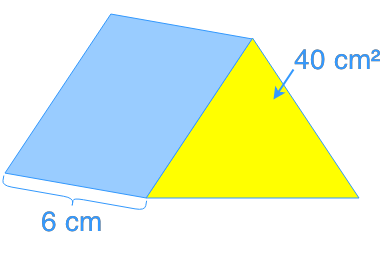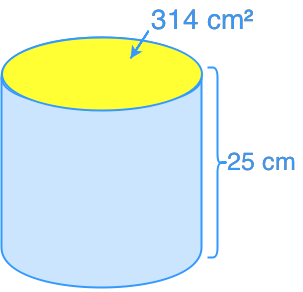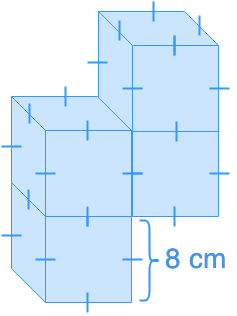# Introduction to volume

### Introduction to volume

In this lesson, we will make use of what we learn from the surface area chapter to calculate the volume of 3D shapes like triangular prisms and cylinders.

#### Lessons

• Introduction
a)
Introduction to Volume
• What is volume?
• Difference between volume and capacity
• What are prisms and pyramids?

• 1.
Find the volume.
a)b)c)• 2.
A triangular prism vase has a base of 64 cm² and a height of 15 cm. If the water level rises by 4 cm after putting in a stone, what is the volume of the stone?